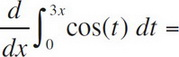# AP Calculus AB Practice Test 4

### Test Information8 questions24 minutes

Calculator Allowed

1.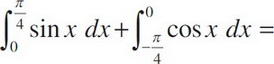2. Boats A and B leave the same place at the same time. Boat A heads due north at 12 km/hr. Boat B heads due east at 18 km/hr. After 2.5 hours, how fast is the distance between the boats increasing (in km/hr)?

3. If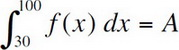and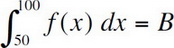, then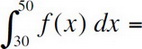4. If f(x) = 3x2 - x, and g(x) = f-1(x), then g′(10) could be

5.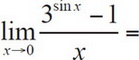6. The volume generated by revolving about the y-axis the region enclosed by the graphs y = 9 - x2 and y = 9 - 3x, for 0 ≤ x ≤ 2, is

7. The average value of the function f(x) = ln2x on the interval [2, 4] is

8.